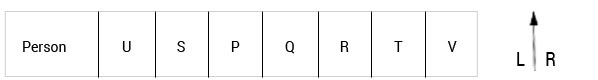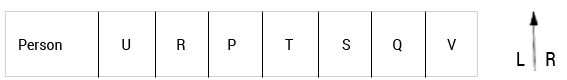# Saint-Gobain Reasoning Question

DIRECTIONS for question 1 to 5 : Read the information given below and answer the question that follows.

Seven people- P, Q, R, S, T, U and V are sitting in a straight line facing north with equal distances between each other but not necessarily in the same order.
Q sits exactly in the middle of the line. R is an immediate neighbor of O. Only three people sit between U and R. S is an immediate neighbor of U. T sits fourth to the right of S. P sits at one of the positions to the left of T.

1. As per the given arrangement, four of the following five are alike in a certain way and hence form a group. Which of the following does not belong to the group?
1. VT
2. RT
3. SU
4. QP
5. PTAs per the given arrangement, PT does not belong to the group, because both persons in all other options are immediate neighbor of each other.
2. Who sits exactly between R and V?
1. P
2. S
3. Q
4. T
5. Other than those given as optionT sits exactly between R and V.
3. What is the position of S with respect to P?
1. Second to the left
2. Second to the right
3. Immediate right
4. Immediate left
5. Third to the rightPosition of S immediate left of P.
4. Who sits third to the left of U?
1. S
2. V
3. Q
4. P
5. No one as U sits at the extreme ends of the lineNo one sits third to the left of U because U sits at the extreme ends of the line.
5. If T and Q interchange their places and so do R and S, who will sit between V and T as per the new arrangement?
1. Both Q and S
2. Only P
3. Both P and S
4. Only U
5. Both S and R
If T and Q interchange their places and so do R and S,According to new arrangement, both Q and S will sit between V and T.
6. 3    9    45    315    ?    31185
1. 2465
2. 2685
3. 2955
4. 2835
5. 2785
The pattern is as follows:
3 × 3 = 9
9 × 5 = 45
45 × 7 = 315
315 × 9 = 2835
7. 12    14    18    26    42 ?
1. 106
2. 74
3. 92
4. 68
5. 84
The pattern is as follows:
12 + 2 = 14
14 + 4 = 18
18 + 8 = 26
26 + 16 = 42
42 + 32 = 74
DIRECTIONS for question 8 to 9: Study the following question and mark the best possible option.

#WU7*C3&Fj4E @ ZL%V8SQA\$N9¥2M65

8. How many such even numbers are there in the given arrangement, each of which is immediately preceded by a symbol and also followed by a letter?
1. One
2. Two
3. None
4. Three
5. More than three
In Β 4 E and ¥ 2 M , 4 and 2 are the Numbers which is immediately preceded by a symbol and also followed by a letter.
9. Which of the following is sixth to the right of the twentieth from the right end of the given arrangement?
1. %
2. Z
3. Β
4. L
5. V